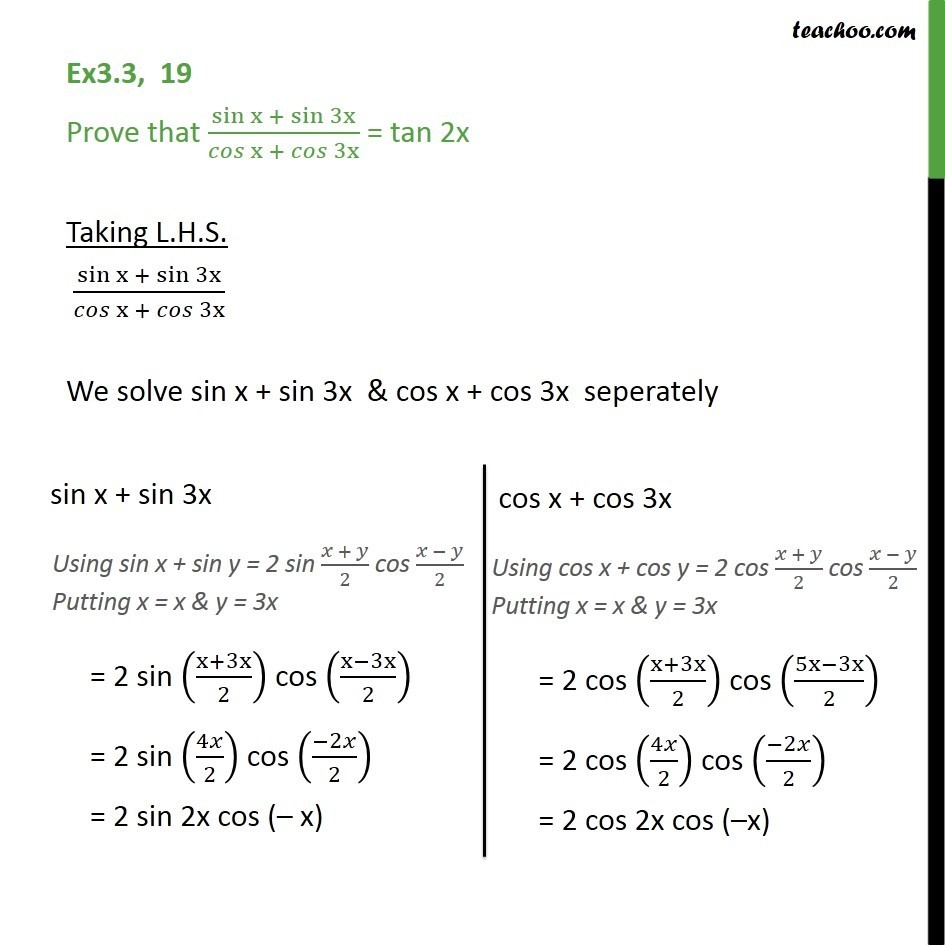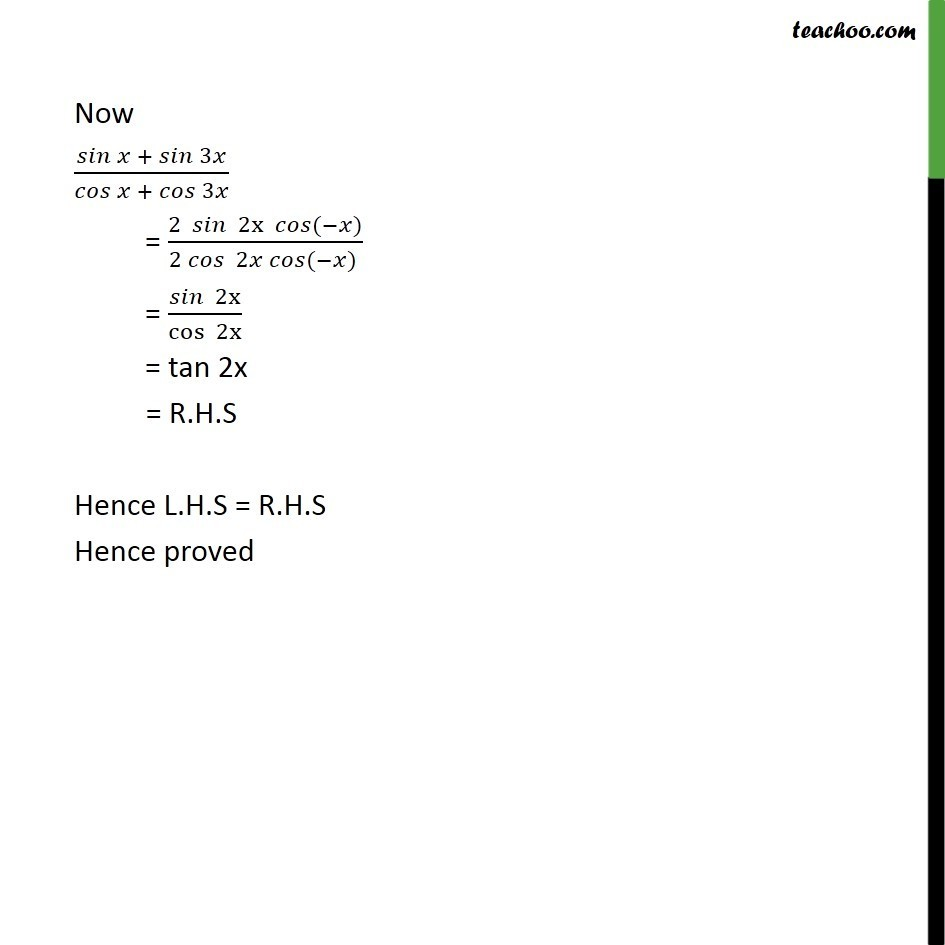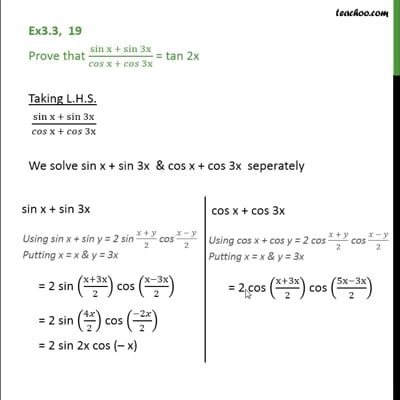Ex 3.3

Chapter 3 Class 11 Trigonometric Functions
Serial order wiseThis video is only available for Teachoo black users

Solve all your doubts with Teachoo Black (new monthly pack available now!)

### Transcript

Ex3.3, 19 Prove that sin x + sin 3x /( x + 3x ) = tan 2x Taking L.H.S. sin x + sin 3x /( x + 3x ) We solve sin x + sin 3x & cos x + cos 3x seperately Now + 3 / + 3 = (2 2x ( ) )/(2 2 ( ) ) = 2x /cos 2x = tan 2x = R.H.S Hence L.H.S = R.H.S Hence proved